## 8.3.2 Smooth Manifolds

The manifold definition given in Section 4.1.2 is often called a topological manifold. A manifold defined in this way does not necessarily have enough axioms to ensure that calculus operations, such as differentiation and integration, can be performed. We would like to talk about velocities on the configuration spaceor in general for a continuous state space. As seen in Chapter 4, the configuration space could be a manifold such as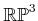. Therefore, we need to define some more qualities that a manifold should possess to enable calculus. This leads to the notion of a smooth manifold.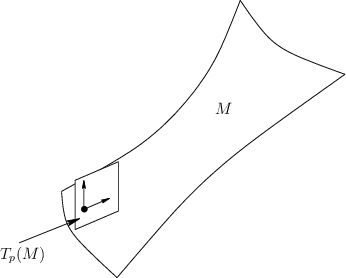Assume thatis a topological manifold, as defined in Section 4.1.2. For example,could represent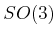, the set of all rotation matrices for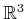. A simpler example that will be helpful to keep in mind is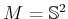, which is a sphere in. We want to extend the concepts of Section 8.3.1 from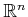to manifolds. One of the first definitions will be the tangent space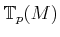at a point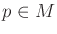. As you might imagine intuitively, the tangent vectors are tangent to a surface, as shown in Figure 8.8. These will indicate possible velocities with which we can move along the manifold from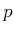. This is more difficult to define for a manifold than forbecause it is easy to express any point inusingcoordinates, and all local coordinate frames for the tangent spaces at every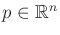are perfectly aligned with each other. For a manifold such as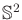, we must define tangent spaces in a way that is not sensitive to coordinates and handles the fact that the tangent plane rotates as we move around on.

First think carefully about what it means to assign coordinates to a manifold. Supposehas dimensionand is embedded in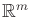. For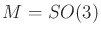,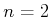and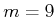. For,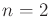and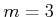. The number of coordinates should be, the dimension of; however, manifolds embedded inare often expressed as a subset offor which some equality constraints must be obeyed. We would like to express some part ofin terms of coordinates in.

Subsections
Steven M LaValle 2020-08-14